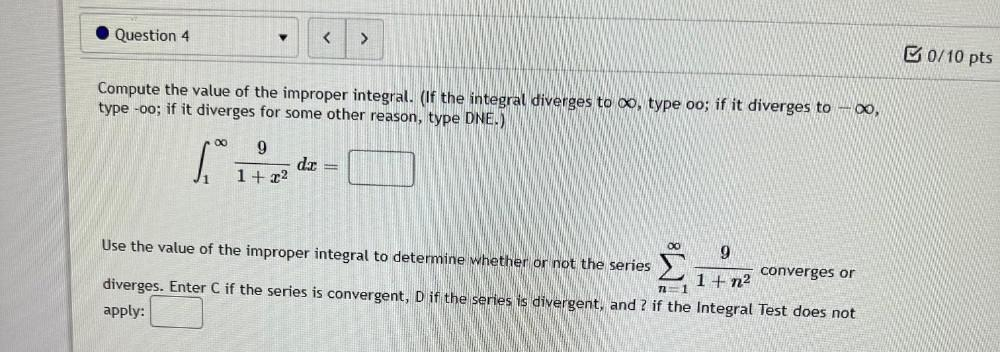Question:

# Question 4 < > B0/10 pts Compute the value of the improper integral. (If the integral diverges to , type oo; if it diverges to –Question 4 < > B0/10 pts Compute the value of the improper integral. (If the integral diverges to , type oo; if it diverges to –®, type -oo; if it diverges for some other reason, type DNE.) 00 9 si dat 1 + x2 Use the value of the improper integral to determine whether or not the series 9 converges or 1 + n2 diverges. Enter C if the series is convergent, D if the series is divergent, and ? if the Integral Test does not apply: 12 = 1